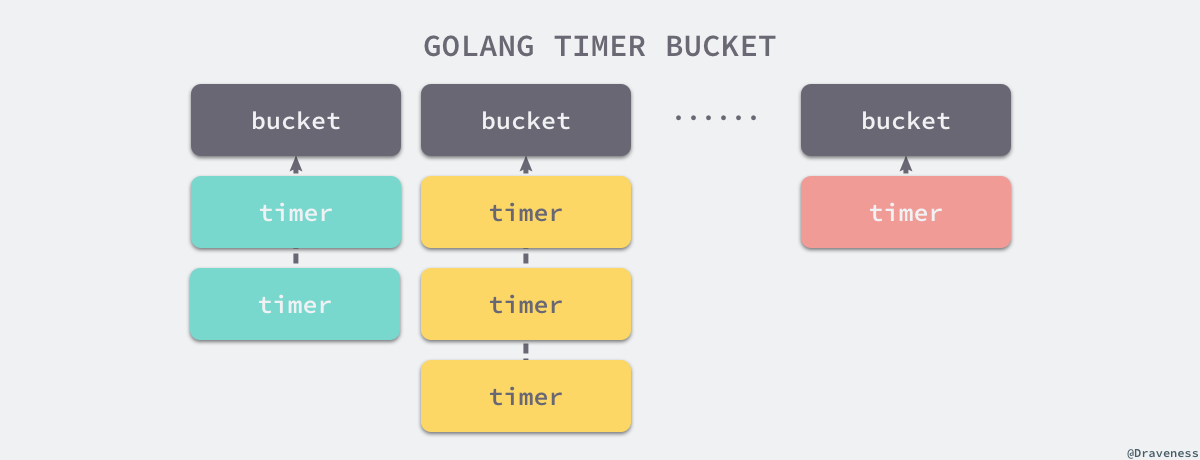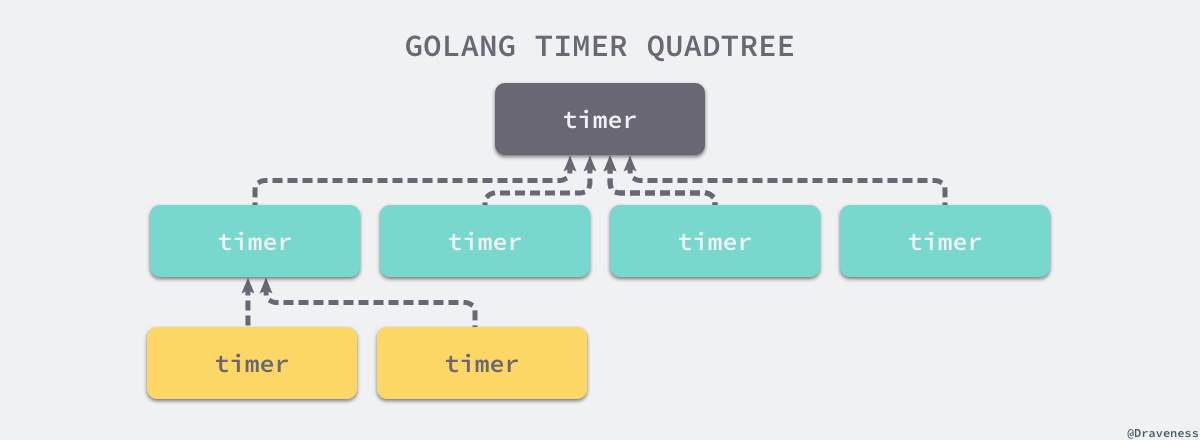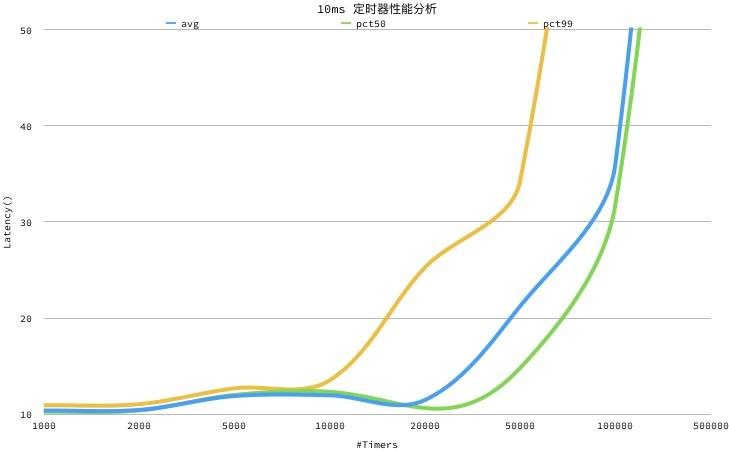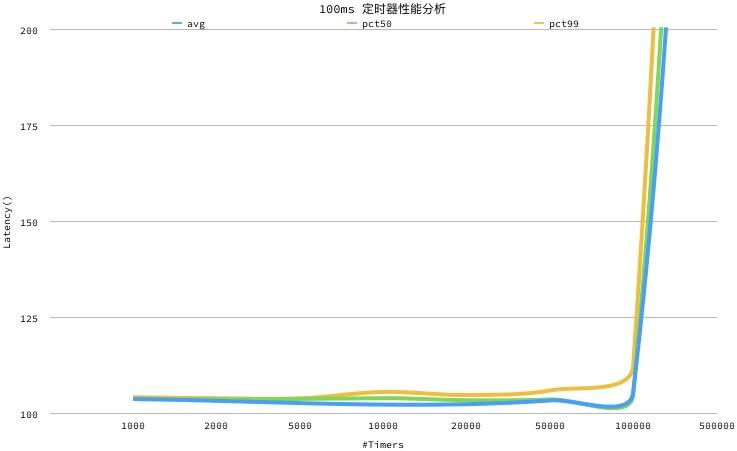# 5.3 定时器

## 1. 结构

`timer` 就是 Golang 定时器的内部表示，每一个 `timer` 其实都存储在堆中，`tb` 就是用于存储当前定时器的桶，而 `i` 是当前定时器在堆中的索引，我们可以通过这两个变量找到当前定时器在堆中的位置：

``````type timer struct {
tb *timersBucket
i  int

when   int64
period int64
f      func(interface{}, uintptr)
arg    interface{}
seq    uintptr
}
``````

`when` 表示当前定时器（Timer）被唤醒的时间，而 `period` 表示两次被唤醒的间隔，每当定时器被唤醒时都会调用 `f(args, now)` 函数并传入 `args` 和当前时间作为参数。然而这里的 `timer` 作为一个私有结构体其实只是定时器的运行时表示，`time` 包对外暴露的定时器使用了如下所示的结构体：

``````type Timer struct {
C <-chan Time
r runtimeTimer
}
``````

`Timer` 定时器必须通过 `NewTimer` 或者 `AfterFunc` 函数进行创建，其中的 `runtimeTimer` 其实就是上面介绍的 `timer` 结构体，当定时器失效时，失效的时间就会被发送给当前定时器持有的 Channel `C`，订阅管道中消息的 Goroutine 就会收到当前定时器失效的时间。

`time` 包中，除了 `timer``Timer` 两个分别用于表示运行时定时器和对外暴露的 API 之外，`timersBucket` 这个用于存储定时器的结构体也非常重要，它会存储一个处理器上的全部定时器，不过如果当前机器的核数超过了 64 核，也就是机器上的处理器 P 的个数超过了 64 个，多个处理器上的定时器就可能存储在同一个桶中：

``````type timersBucket struct {
lock         mutex
gp           *g
created      bool
sleeping     bool
rescheduling bool
sleepUntil   int64
waitnote     note
t            []*timer
}
``````## 2. 工作原理

### 2.1. 创建

`time` 包对外提供了两种创建定时器的方法，第一种方法就是 `NewTimer` 接口，这个接口会创建一个用于通知触发时间的 Channel、调用 `startTimer` 方法并返回一个创建指向 `Timer` 结构体的指针：

``````func NewTimer(d Duration) *Timer {
c := make(chan Time, 1)
t := &Timer{
C: c,
r: runtimeTimer{
when: when(d),
f:    sendTime,
arg:  c,
},
}
startTimer(&t.r)
return t
}
``````

``````func AfterFunc(d Duration, f func()) *Timer {
t := &Timer{
r: runtimeTimer{
when: when(d),
f:    goFunc,
arg:  f,
},
}
startTimer(&t.r)
return t
}
``````

`startTimer` 基本上就是创建定时器的入口了，所有定时器的创建和重启基本上都需要调用该函数：

``````func startTimer(t *timer) {
}

tb := t.assignBucket()
}
``````

``````func (tb *timersBucket) addtimerLocked(t *timer) bool {
t.i = len(tb.t)
tb.t = append(tb.t, t)
if !siftupTimer(tb.t, t.i) {
return false
}
if t.i == 0 {
if tb.sleeping && tb.sleepUntil > t.when {
tb.sleeping = false
notewakeup(&tb.waitnote)
}
if tb.rescheduling {
tb.rescheduling = false
}
if !tb.created {
tb.created = true
go timerproc(tb)
}
}
return true
}
``````

`addtimerLocked` 会先将最新加入的定时器加到队列的末尾，随后调用 `siftupTimer` 将当前定时器与四叉树（或者四叉堆）中的父节点进行比较，保证父节点的到期时间一定小于子节点：### 2.2. 触发

``````func timerproc(tb *timersBucket) {
tb.gp = getg()
for {
tb.sleeping = false
now := nanotime()
delta := int64(-1)

// inner loop

if delta < 0 {
tb.rescheduling = true
goparkunlock(&tb.lock, waitReasonTimerGoroutineIdle, traceEvGoBlock, 1)
continue
}
tb.sleeping = true
tb.sleepUntil = now + delta
noteclear(&tb.waitnote)
notetsleepg(&tb.waitnote, delta)
}
}
``````

``````func notetsleepg(n *note, ns int64) bool {
gp := getg()
if gp == gp.m.g0 {
throw("notetsleepg on g0")
}
semacreate(gp.m)
entersyscallblock()
ok := notetsleep_internal(n, ns, nil, 0)
exitsyscall()
return ok
}
``````

1. 如果桶中不包含任何定时器就会直接返回并陷入休眠等待定时器加入当前桶；
2. 如果四叉树最上面的定时器还没有到期会通过 `notetsleepg` 方法陷入休眠等待最近定时器的到期；
3. 如果四叉树最上面的定时器已经到期；
• 当定时器的 `period > 0` 就会设置下一次会触发定时器的时间并将当前定时器向下移动到对应的位置；
• 当定时器的 `period <= 0` 就会将当前定时器从四叉树中移除；
4. 在每次循环的最后都会从定时器中取出定时器中的函数、参数和序列号并调用函数触发该计数器；
``````        for {
if len(tb.t) == 0 {
delta = -1
break
}
t := tb.t
delta = t.when - now
if delta > 0 {
break
}
ok := true
if t.period > 0 {
t.when += t.period * (1 + -delta/t.period)
if !siftdownTimer(tb.t, 0) {
ok = false
}
} else {
last := len(tb.t) - 1
if last > 0 {
tb.t = tb.t[last]
tb.t.i = 0
}
tb.t[last] = nil
tb.t = tb.t[:last]
if last > 0 {
if !siftdownTimer(tb.t, 0) {
ok = false
}
}
t.i = -1 // mark as removed
}
f := t.f
arg := t.arg
seq := t.seq
f(arg, seq)
}
``````

### 2.3. 休眠

``````func timeSleep(ns int64) {
if ns <= 0 {
return
}

gp := getg()
t := gp.timer
if t == nil {
t = new(timer)
gp.timer = t
}
*t = timer{}
t.when = nanotime() + ns
t.arg = gp
tb := t.assignBucket()
lock(&tb.lock)
unlock(&tb.lock)
}
goparkunlock(&tb.lock, waitReasonSleep, traceEvGoSleep, 2)
}
``````

`timeSleep` 会创建一个新的 `timer` 结构体，在初始化的过程中我们会传入当前 Goroutine 应该被唤醒的时间以及唤醒时需要调用的函数 `goroutineReady`，随后会调用 `goparkunlock` 将当前 Goroutine 陷入休眠状态，当定时器到期时也会调用 `goroutineReady` 方法唤醒当前的 Goroutine：

``````func goroutineReady(arg interface{}, seq uintptr) {
}
``````

`time.Sleep` 方法其实只是创建了一个会在到期时唤醒当前 Goroutine 的定时器并通过 `goparkunlock` 将当前的协程陷入休眠状态等待定时器触发的唤醒。

### 2.4. Ticker

``````type Ticker struct {
C <-chan Time // The channel on which the ticks are delivered.
r runtimeTimer
}
``````

``````func NewTicker(d Duration) *Ticker {
if d <= 0 {
panic(errors.New("non-positive interval for NewTicker"))
}
c := make(chan Time, 1)
t := &Ticker{
C: c,
r: runtimeTimer{
when:   when(d),
period: int64(d),
f:      sendTime,
arg:    c,
},
}
startTimer(&t.r)
return t
}

func Tick(d Duration) <-chan Time {
if d <= 0 {
return nil
}
return NewTicker(d).C
}
``````

`Tick` 其实也只是对 `NewTicker` 的简单封装，从实现上我们就能看出来它其实就是调用了 `NewTicker` 获取了计时器并返回了计时器中 Channel，两个创建计时器的方法的实现都并不复杂而且费容易理解，所以在这里也就不详细展开介绍了。

## 3. 性能分析

``````func runTimers(count int) {
durationCh := make(chan time.Duration, count)

wg := sync.WaitGroup{}
for i := 0; i < count; i++ {
go func() {
startedAt := time.Now()
time.AfterFunc(10*time.Millisecond, func() {
defer wg.Done()
durationCh <- time.Since(startedAt)
})
}()

}
wg.Wait()

close(durationCh)

durations := []time.Duration{}
totalDuration := 0 * time.Millisecond
for duration := range durationCh {
durations = append(durations, duration)
totalDuration += duration
}
averageDuration := totalDuration / time.Duration(count)
sort.Slice(durations, func(i, j int) bool {
return durations[i] < durations[j]
})

fmt.Printf("run %v timers with average=%v, pct50=%v, pct99=%v\n", count, averageDuration, durations[count/2], durations[int(float64(count)*0.99)])
}
``````

``````\$ go test ./... -v
=== RUN   TestTimers
run 1000 timers with average=10.367111ms, pct50=10.234219ms, pct99=10.913219ms
run 2000 timers with average=10.431598ms, pct50=10.37367ms, pct99=11.025823ms
run 5000 timers with average=11.873773ms, pct50=11.986249ms, pct99=12.673725ms
run 10000 timers with average=11.954716ms, pct50=12.313613ms, pct99=13.507858ms
run 20000 timers with average=11.456237ms, pct50=10.625529ms, pct99=25.246254ms
run 50000 timers with average=21.223818ms, pct50=14.792982ms, pct99=34.250143ms
run 100000 timers with average=36.010924ms, pct50=31.794761ms, pct99=128.089527ms
run 500000 timers with average=176.676498ms, pct50=138.238588ms, pct99=676.967558ms
--- PASS: TestTimers (1.21s)
``````• 定时器触发的时间一定会晚于创建时传入的时间，假设定时器需要等待 10ms 触发，那它触发的时间一定是晚于 10ms 的；
• 当并发的定时器数量达到 5000 时，定时器的平均误差达到了 ~18%，99 分位数上的误差达到了 ~26%；
• 并发定时器的数量超过 5000 之后，定时器的误差就变得非常明显，不能有效、准确地完成计时任务；## 4. 总结

Go 语言的定时器在并发编程起到了非常重要的作用，它能够为我们提供比较准确的相对时间，基于它的功能，标准库中还提供了计时器、休眠等接口能够帮助我们在 Go 语言程序中更好地处理过期和超时等问题。

## 6. 其他

### 6.2. 微信公众号